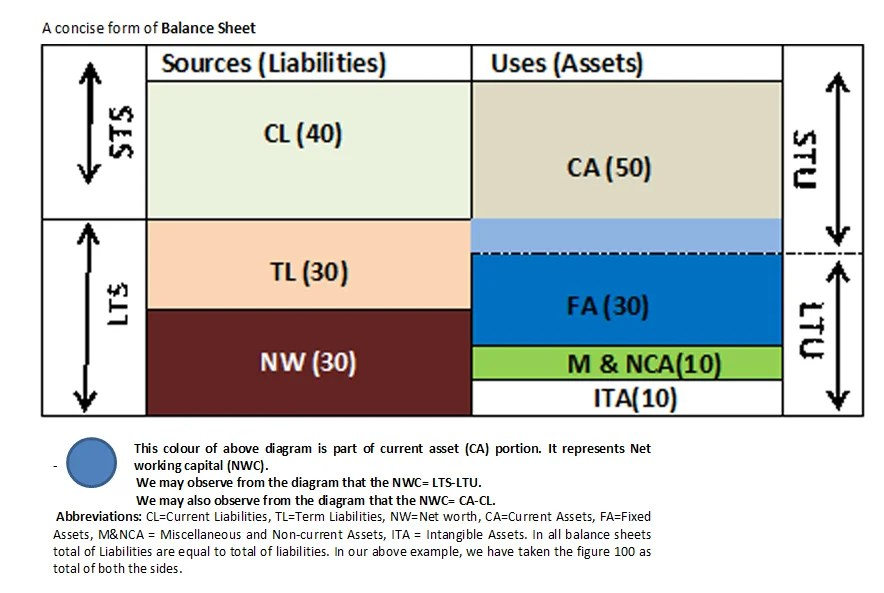Financial AnalysisThe working capital gap in simple words is the difference between total current assets and total current liabilities other than bank. It can also be defined as Long term sources less long term uses.

Working capital gap= Current assets – current liabilities (other than bank borrowings)
For example,Currrent if current asset is 100 and current liabilities is 80,bank liability is 20. Then, current liability other than bank borrowings is 80-20=60. The working capital gap is 100-60=40

The net capital gap is long term sources of the company less long term uses of the company. It means, the surplus in long term sources like owners capital and term loans less long term uses like Fixed Assets, miscellaneous and Non-current Assets, Intangible Assets.

For example, if the total liability of a company is Rs.100 lakh comprising Rs.40 lakh as current liability and Rs.60 lakh as long term liabilty (including owners equity). It means totla sources of the company which can be used to acquire various types of assets is Rs.100 lakhs.

Now let us presume that the company has used funds from above source as under;

Rs. 50 lakh  used for buying current assets and  Rs.50 lakh used for acquiring Fixed Assets, miscellaneous assets, Non-current Assets and Intangible Assets. It means the company has used only Rs.50 lakh from its long term liability (source) of Rs.60 lakh to its long term uses (Rs.50 lakh). Thus, Rs.10 lakh (60-50) from long term liability (source) is available with the company for short term uses.

Working capital gap= Current assets (50) less current liabilities(40) i.e. 50-40= 10
or working capital gap =Long term sources less long term uses i.e. 60-50=10

Illustration by diagram:

The structure of long-term sources (LTS), short term source (STS), long- term uses(LTU) and short-term uses(STU), Net-Working capital is explained in the following diagram of a balance sheet.Abbreviations: CL=Current Liabilities, TL=Term Liabilities, NW=Net worth, CA=Current Assets, FA=Fixed Assets, M&NCA = Miscellaneous and Non-current Assets, ITA = Intangible Assets. In all balance- sheets total of Liabilities is equal to the total of liabilities. In our above example, we have taken the figure 100 as the total of both the sides.

Examples of the long-term source (LTS) of funds are funds from operation (retained profit), Share capital, the issue of debentures, long-term investments, the  sale of fixed assets and sale proceeds of long-term investments etc.

Examples of long-term use (LTU) of funds are the purchase of fixed assets, repayment of long-term loans, repayment of debentures and bonds, the redemption of preferential shares, loss from business operations etc.

Related article: What is operating cycle of a manufacturing unit?